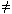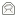## Introduction

This page explains some of the maths concepts that RSA is based on, and then provides a complete proof that RSA works correctly. However, there is no mathematical proof that RSA is secure, everyone takes that on trust! This is fairly advanced material and not necessary to understand the use and applications of the algorithm.

## Modular ArithmeticRSA uses modular arithmetic. This is similar to conventional arithmetic, but only uses positive integers that are less than a chosen value, called the modulus. Addition, subtraction and multiplication work like regular maths, but there is no division. You can use any value for the modulus; the diagram uses 13, so counting goes 0, 1, 2, ..., 11, 12, 0, 1, 2 ... The notation used for expressions involving modular arithmetic is:

x = y (mod m)

Which reads as "x is equivalent to y, modulo m". What this means is that x and y leave the same remainder when divided by m. For example, 7 = 23 (mod 8) and 22 = 13 (mod 9). The following statement is a basic principle of modular arithmetic:

a + kp = a (mod p)

You can visualise this on the diagram - each time you add p you go round the circle, back to where you started. It doesn't matter where you start, how big the circle is, or how many times you do it, it's always true.

## Primality and Coprimality

• A number is prime if the only numbers that exactly divide it are 1 and itself. e.g. 17 is prime, but 15 isn't, because it's divisible by 3 and 5.
• A pair of numbers are coprime if the largest number that exactly divides both of them is 1. The numbers themselves don't have to be prime. e.g. 8 and 9 are coprime, but 8 and 10 are not, because they're both divisible by 2.
• If you have a pair of distinct prime numbers, they will always be coprime to each other.

## Chinese Remainder Theorem

This theorem provides a way to combine two modular equations that use different moduli.

 Theorem Proof x = y (mod p) x = y (mod q) with p and q coprimex = y (mod pq) x = y (mod p)x = y + kpx - y = kpp divides (x - y) by a similar route, q divides (x - y) as p and q are coprime, pq divides (x - y)x - y = l(pq)x = y (mod pq)

## Fermat/Euler Theorem

This theorem is a surprising identity that relates the exponent to the modulus.

Theorem Proof xp-1 = 1 (mod p) if p is prime and x0 (mod p) consider the set Q, of numbers 1, 2, ... p-1 as p is prime, these numbers are coprime to p 0 is not coprime to pQ includes all the numbers in (mod p) coprime to p now consider the set U, obtained by multiplying each element of Q by x (mod p) both x and each element of Q are coprime to peach element of U is coprime to p also, each element of U is distinct, which we prove by contradiction start by assuming two elements are not distinct: xQi = xQj (mod p) with ijQi = Qj (mod p) as x0 but elements of Q are distinct, so this is a contradictionelements of U are distinct U uses all the numbers in (mod p) that are coprime to p, just like QU is a permutation of QU1.U2 ... Up-1 = Q1.Q2 ... Qp-1 (mod p)xQ1.xQ2 ... xQp-1 = Q1.Q2 ... Qp-1 (mod p) and if we cancel Q1.Q2 ... Qp-1xp-1 = 1 (mod p)

## RSA Correctness

Here we prove that the combined process of encrypting and decrypting a message correctly results in the original message.

Theorem Proof C = Me (mod n) M' = Cd (mod n)M' = M (mod n) where (d, e, n) is a valid RSA key, with n = pq and 0 < M < minimum(p,q) first we combine the two exponentiations: M' = Med (mod n) d and e are generated so that de = k(p-1)(q-1) + 1M' = Mk(p-1)(q-1) + 1 (mod n)M' = M.Mk(p-1)(q-1) (mod n)     * consider X = Mk(p-1)(q-1) (mod p)X = (M(p-1))k(q-1) (mod p) the Fermat/Euler theorem tells us that M(p-1) = 1 (mod p)X = 1k(q-1) (mod p)X = 1 (mod p) by a similar route, X = 1 (mod q) as p and q are distinct primes, we can combine these with the Chinese remainder theoremX = 1 (mod pq)Mk(p-1)(q-1) = 1 (mod n) finally, we substitute this back into the equation marked *M' = M.1 (mod n)M' = M (mod n)
© 1998 - 2012Paul Johnston, distributed under the BSD License   Updated:13 Jul 2009